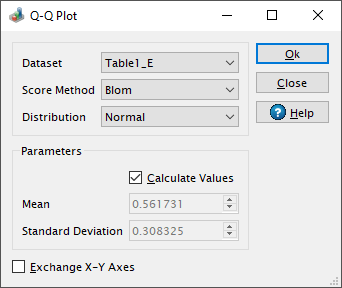# Q-Q Plot Dialog

This dialog is activated by selecting the Q-Q Plot... command from the Plot -> Statistical Graphs menu. It can be used in order to create and customize a Q-Q (quantile-quantile) plot of the selected data column in the active table window.

Figure 5-111. The Frequency Count dialog.The N valid input data values xi are first sorted ascendingly: x1x2 ≤ ... ≤ xN. After that the index i of the ordered values is used to calculate a score (s) using one of the five methods listed below:

Blom

(default): s = (i - 0.375)/(N + 0.25)

Benard

s = (i - 0.3)/(N + 0.4)

Hazen

s = (i - 0.5)/N

VanDerWaerden

s = i/(N + 1)

KaplanMeier

s = i/N

The sorted values are represented on the Q-Q plot by points whose X coordinates are the xi values and whose Y coordinates are calculated using the inverse of the cumulative distribution functions of the s scores calculated above.

There are five distributions available for Q-Q plots and the inverse of their cumulative distribution functions are calculated using the following formulas (available with muParser as script engine):

Normal

(default): normalinv(s, m, sd), where m is the arithmetic mean and sd is the standard deviation of the input data set.

Lognormal

logninv(s, scale, shape)

Exponential

expinv(s, m), where m is the arithmetic mean of the input data set.

Weibull

wblinv(s, scale, shape)

Gamma

gaminv(s, shape, scale)

The scale and shape parameters that are used for the Lognormal, Weibull and Gamma distributions are calculated using the maximum likelihood estimation (MLE) method.